# Mathematics Support

Math 7 | Unit 1 2 3 4 5 6 7 8 9

### Angles, Triangles, and Prisms

Angle Relationships

Drawing Polygons with Given Conditions

Solid Geometry

## Angle Relationships

This week your student will be working with some relationships between pairs of angles.

• If two angles add to 90, then we say they are complementary angles. If two angles add to 180, then we say they are supplementary angles.  For example, angles JGF and JGH below are supplementary angles, because 30+150=180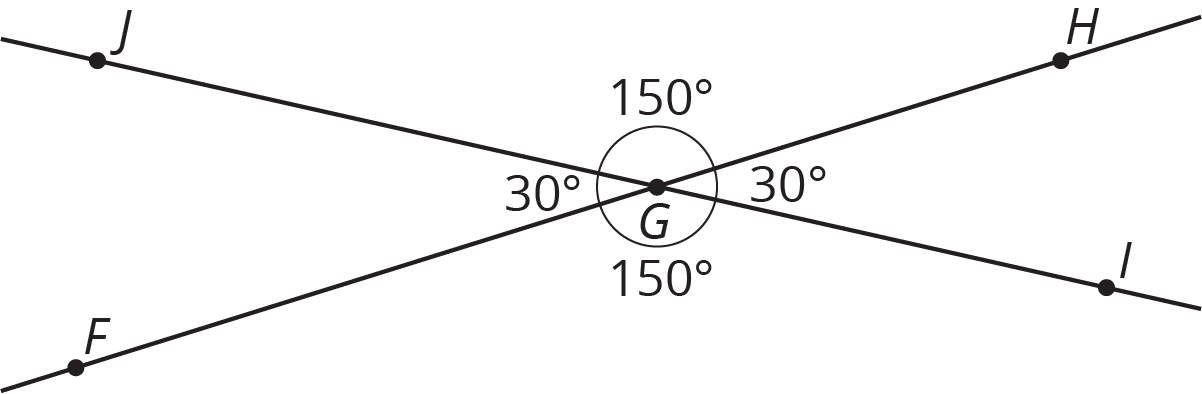• When two lines cross, they form two pairs of vertical angles across from one another. In the previous figure, angles JGF and HGI are vertical angles. So are angles JGH and FGJ. Vertical angles always have equal measures.

Here is a task to try with your student:  Rectangle PQRS has points T and Von two of its sides.1. Angles SVT and TVR are supplementary. If angle SVT measures 117, what is the measure of angle TVR?
2. Angles QTP and QPT are complementary. If angle QTP measures 53, what is the measure of angle QPT?

Solution:

1. Angle TVR measures 63, because 180117=63.
2. Angle QPT measures 37, because 9053=37.

## Drawing Polygons with Given Conditions

This week your student will be drawing shapes based on a description. What options do we have if we need to draw a triangle, but we only know some of its side lengths and angle measures?

• Sometimes we can draw more than one kind of triangle with the given information. For example, “sides measuring 5 units and 6 units, and an angle measuring 32” could describe two triangles that are not identical copies of each other.• Sometimes there is only one unique triangle based on the description. For example, here are two identical copies of a triangle with two sides of length 3 units and an angle measuring 60. There is no way to draw a different triangle (a triangle that is not an identical copy) with this description.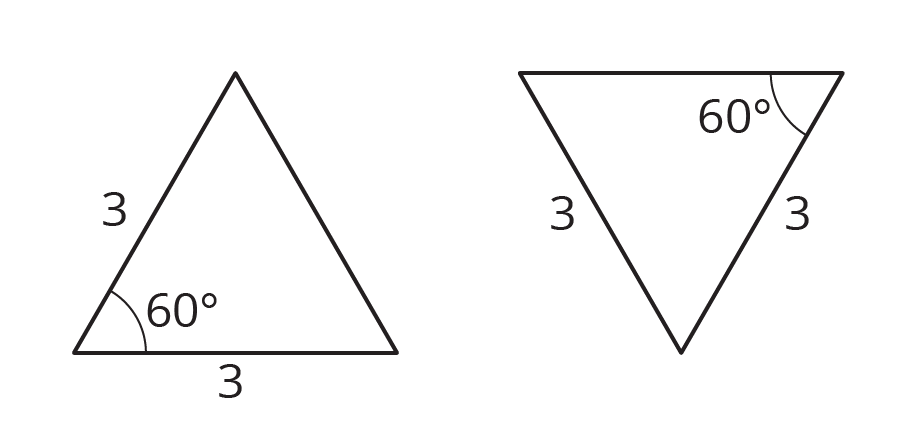• Sometimes it is not possible to draw a triangle with the given information. For example, there is no triangle with sides measuring 4 inches, 5 inches, and 12 inches. (Try to draw it and see for yourself!)

Here is a task to try with your student:  Using each set of conditions, can you draw a triangle that is not an identical copy of the one shown?

1. A triangle with sides that measure 4, 6, and 9 units.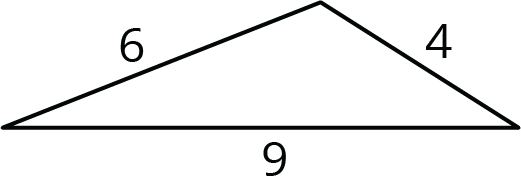2. A triangle with a side that measures 6 units and angles that measure 45and 90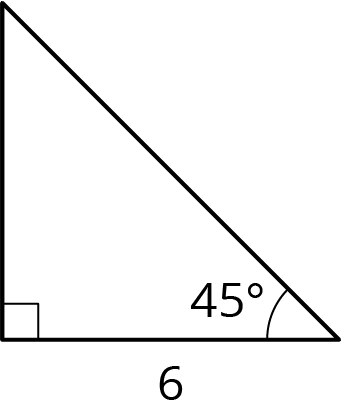Solution:

1. There is no way to draw a different triangle with these side lengths. Every possibility is an identical copy of the given triangle. (You could cut out one of the triangles and match it up exactly to the other.) Here are some examples: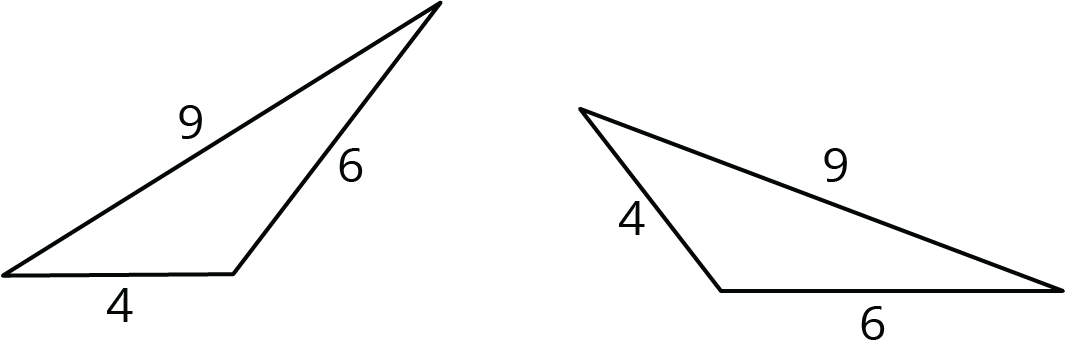2. You can draw a different triangle by putting the side that is 6 opposite from the 90 angle instead of next to it. This is not an identical copy of the given triangle, because it is smaller.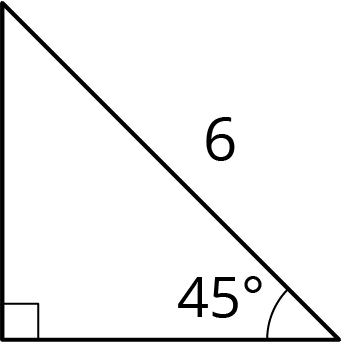## Solid Geometry

This week your student will be thinking about the surface area and volume of three-dimensional figures. Here is a triangular prism. Its base is a right triangle with sides that measure 12, 12, and 17 inches.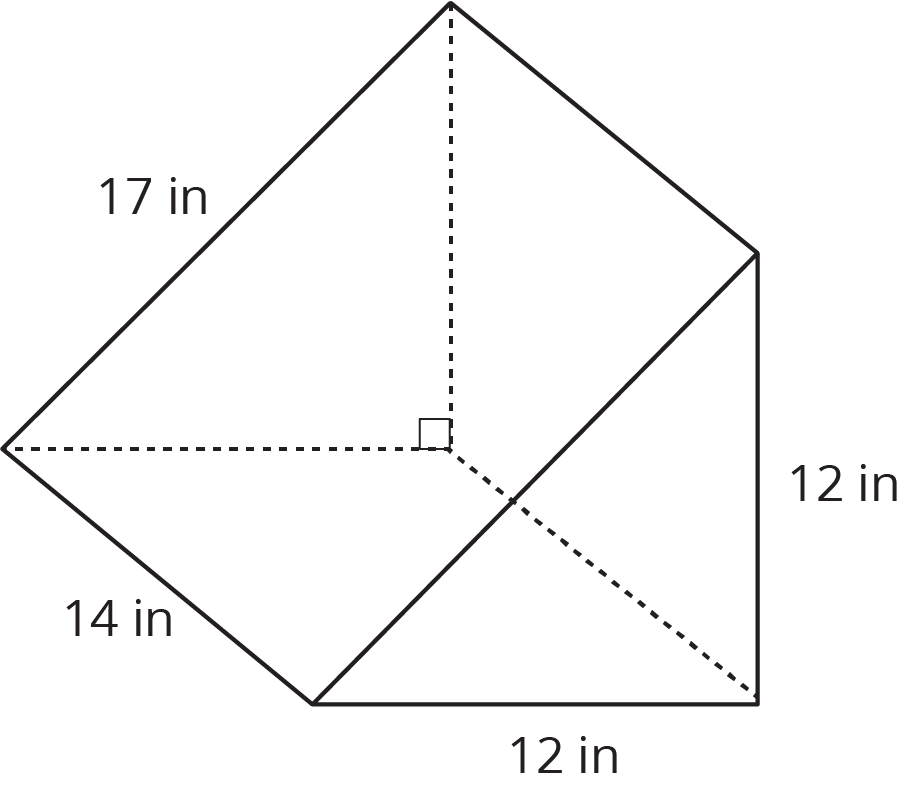In general, we can find the volume of any prism by multiplying the area of its base times its height.  For this prism, the area of the triangular base is 72 in2, so the volume is 7214, or 1,008 in3.

To find the surface area of a prism, we can find the area of each of the faces and add them up. The example prism has two faces that are triangles and three faces that are rectangles. When we add all these areas together, we see that the prism has a total surface area of 72+72+168+168+238, or 718 in2.

Here is a task to try with your student:  The base of this prism is a hexagon where all the sides measure 5 cm. The area of the base is about 65 cm2.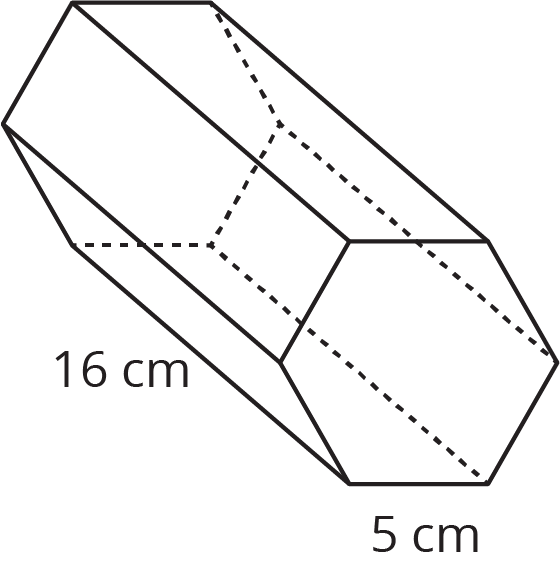1. What is the volume of the prism?
2. What is the surface area of the prism?

Solution:

1. The volume of the prism is about 1,040 cm3, because 6516=1,040.
2. The surface area of the prism is 610 cm2, because 165=80 and 65+65+80+80+80+80+80+80=610.

IM 6–8 Math was originally developed by Open Up Resources and authored by Illustrative Mathematics, and is copyright 2017-2019 by Open Up Resources. It is licensed under the Creative Commons Attribution 4.0 International License (CC BY 4.0). OUR's 6–8 Math Curriculum is available at https://openupresources.org/math-curriculum/.

Disclaimer: This site provides external links and videos as a convenience and for informational purposes only. The appearance of external hyperlinks on the MCPS Family Mathematics Support Center website does not constitute an endorsement by the Montgomery County Public School System of any of the products or opinions contained therein.